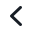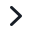# My Math Checker

### Try the automatic checker!Check if the example is correctly calculated

Then replace it with your own calculation.

# Derivatives

Check if the derivatives example to the left is calculated correctly. You can exchange it with your own calculation, and check that one as well.

# Use the checker always when practicing math

### Checking for Middle School, High School and University Level Math

Whether you are a middle school, high school or university level student, or an adult refreshing your memory, 4f Checker helps you to check if your derivation is mathematically correct.

The checker formulates each step in the derivation as a mathematical theorem, and sends the theorem to an automatic theorem prover that runs in the cloud. The derivation is correct, if each step in the derivation is proved correct.

### Trust yourself

In math, there are many ways to get to a solution. Math operations can be made in different order, calculations in several or few steps. We all think in different ways, and it may be difficult to see it the way your teacher does and teaches you. This doesn't mean that your way is wrong. With the checker you can solve assignments your way, and check if it is mathematically correct.

### Subscription details

The monthly subscription My Math Checker includes digital notebooks where you can calculate all your assignments and check the correctness of your calculations. The checker goes through the solution step by step and points out the weak spots and potential problems in your mathematical arguments.

My Math Checker subscription gives you